Orginal Article

# 基于迭代法的非线性弹性均质化研究

1湖南大学机械与运载工程学院, 长沙 410006
2河北工业大学机械工程学院, 天津 300401;

# STUDY ON NONLINEAR ELASTIC HOMOGENIZATION WITH ITERATIVE METHOD

Hou Shujuan1*, Liang Huiyan1, Wang Quanzhong1, Han Xu12

1College of Mechanical and Vehicle Engineering, Hunan University, Changsha 410006, China
2College of Mechanical Engineering, Hebei University of Technology, Tianjin 300401, China;

Abstract

Microstructure is critical to affect or change the macroscopic mechanical properties of composites, and the desired material properties can be obtained by rationally designing the composite microstructure. As an effective design method, homogenization method is used to obtain and design the macro-mechanical properties on the basis of microstructure. However, once considering the nonlinear factors, the realization of homogenization can be very difficult. Therefore, this paper focuses on the nonlinear elastic homogenization of composite materials by theoretical deduction, and solves the problem by direct iteration method. In this study, the equation of nonlinear elastic homogenization is deduced by the asymptotic expansion homogenization method. The iterative steps of direct iteration method are given to solve the nonlinear elastic homogenization equation. According to the iterative steps and the nonlinear elastic homogenization equation, the program in MATLAB language is obtained. The porous materials with three typical constitutive relations are chosen to be the study object. The program and iterative method is verified by comparing the strain energy, maximum displacement and equivalent Poisson’s ratio with the results of detailed model. Then, the application of nonlinear elastic homogenization method is extended to three-dimensional composite materials with multi-scale periodic microstructure, a three-element rubber-based composite material. It is divided into core-scale and layer-scale and homogenized with multi-scale homogenization method. The equivalent elasticity modulus of the core-scale are obtained by linear elastic homogenization method and used as a parameter of a component in layer-scale. Then, the nonlinear elastic homogenization method is used for layer-scale. The macroscopic equivalent performance of the material is obtained and compared with experimental results. The nonlinear elastic homogenization method has certain guiding significance and reference value for the nonlinear homogenization and microstructure design of the composite material.

Keywords： nonlinear elastic ; homogenization ; asymptotic homogenization method ; iteration ; multi-scale ; periodic composite materials

0

Hou Shujuan, Liang Huiyan, Wang Quanzhong, Han Xu. STUDY ON NONLINEAR ELASTIC HOMOGENIZATION WITH ITERATIVE METHOD[J]. Acta Mechanica Sinica, 2018, 50(4): 837-846 https://doi.org/10.6052/0459-1879-18-039

## 1 非线性弹性均质化

### 1.1 非线性弹性均质化理论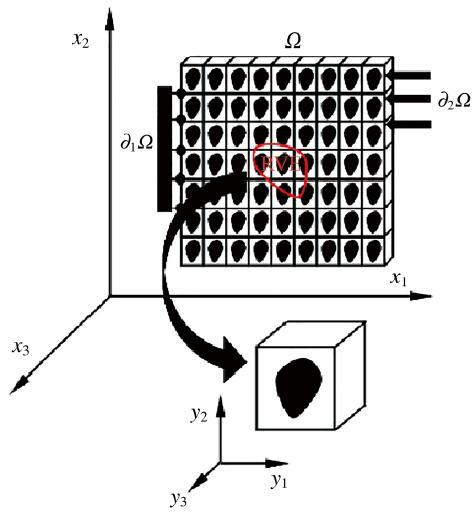Fig.1   Periodic composite materials and RVE

$∂∂xε=∂∂x+1ε∂∂y$

$ui(x,y)=ui(0)(x,y)+εui(1)(x,y)+ε2ui(2)(x,y)$

$εij=ε-1εij(0)+ε0εij(1)+εεij(2)+O(ε2)$

$εij(0)=12(∂ui(0)∂yj+∂uj(0)∂yi)εij(1)=12(∂ui(0)∂xj+∂uj(0)∂xi+∂ui(1)∂yj+∂uj(1)∂yi)εij(2)=12(∂ui(1)∂xj+∂uj(1)∂xi+∂ui(2)∂yj+∂uj(2)∂yi)$

$σij=ε-1σij(0)+ε0σij(1)+εσij(2)+O(ε2)$

$ε(-2)∂σij(0)∂yj+ε(-1)(∂σij(0)∂xj+∂σij(1)∂yj)+ε(0)(∂σij(1)∂xj+∂σij(2)∂yj+fi)+L=0$

$ε(-2):∂σij(0)∂yj=0$

$ε(-1):∂σij(0)∂xj+∂σij(1)∂yj=0$

$ε(0):∂σij(1)∂xj+∂σij(2)∂yj+fi=0$

$σij(0)=σij(0)(x)$

$σij(0)(x)=12Dijkl(ε)(∂uk(0)∂yl+∂ul(0)∂yk)=12[Dij11(ε)(2∂u1(0)∂y1)+Dij12(ε)(∂u1(0)∂y2+∂u2(0)∂y1)+Dij21(ε)(∂u2(0)∂y1+∂u1(0)∂y2)+Dij22(ε)(2∂u2(0)∂y2)]=Dij11(ε)∂u1(0)∂y1+Dij12(ε)∂u1(0)∂y2+Dij21(ε)∂u2(0)∂y1+Dij22(ε)∂u2(0)∂y2=Dijkl(ε)∂uk(0)∂yl$

$σij(0)(x)=0,∂ui(0)∂yj=0$

$∂σij(1)∂yj=∂∂yj[Dijkl(ε)(∂uk(0)∂xl+∂uk(1)∂yl)]=0$

$ui(1)=-χikl∂uk(0)∂xl$

$∂∂yi[Dijkl(ε)(Iklmn-∂χkmn∂yl)]=0$

$DijmnH(ε)=1|Y|∫Y‍Dijkl(ε)(Iklmn-∂χkmn∂yl)dY$

### 1.2 非线性弹性均质化实现

$DijmnH=1|Y|∫Y‍Dijkl(Iklmn-∂χkmn∂yl)dY$

$∫Y‍Dijkl∂χkmn∂yl∂vi∂yjdY=∫Y‍Dijmn∂vi∂yjdY,∀vi∈ṼY$

$χ=NIuI$

$DH=1|Y|∫Y‍(I-BIuI)TD(I-BIuI)dY$

$DH=1|Y|(BI-1I-uI)TK(BI-1I-uI)$

$ε0(11)=100,ε0(22)=010,ε0(12)=001$

$DH=1|Y|∫Y‍(I-BIuI)TD(ε)(I-BIuI)dY$

(1) 给定一个初始应变 $ε(0)$, 计算初始割线弹性矩阵 $D(0),n=0$

(2) 计算等效弹性矩阵 $D(n)H$

$D(n)H=1|Y|∫Y‍(I-BIuI)TD(n)(I-BIuI)dY$

(3) 计算第 $n$次近似应变 $ε(n+1)$

$ε(n+1)=ε̅$

(4) 判断收敛条件, 如果收敛, 停止迭代, 输出结果; 否则, 转到步骤(2), 继续进行迭代.

## 2 二维非线性弹性问题

### 2.1 材料本构关系

Table 1   The constitutive relation expression

Constitutive2*Equation
relation
power function$σ=2ε1.5$
bilinear function$σ=0.2ε,ε„0.010.1ε+0.001,ε>0.01$
exponential2*$σ=0.3(eε-1)$
function

### 2.2 均质化模型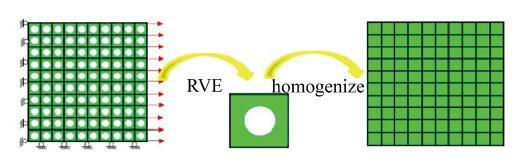Fig.2   Porous material structure

$v'=-εyεx$

### 2.3 均质化结果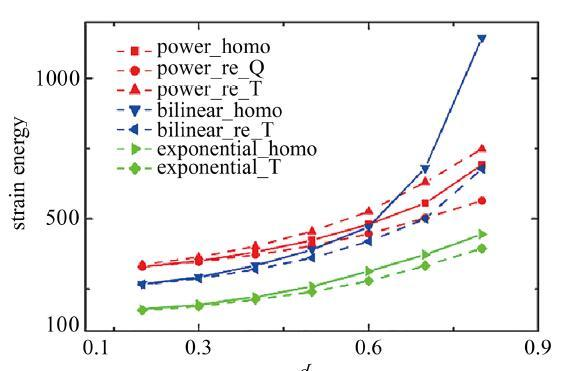Fig.3   The strain energy of porous material structure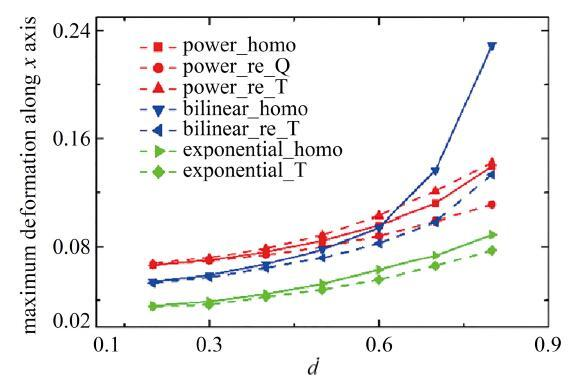Fig.4   The maximum deformation along $x$ axis of porous material structure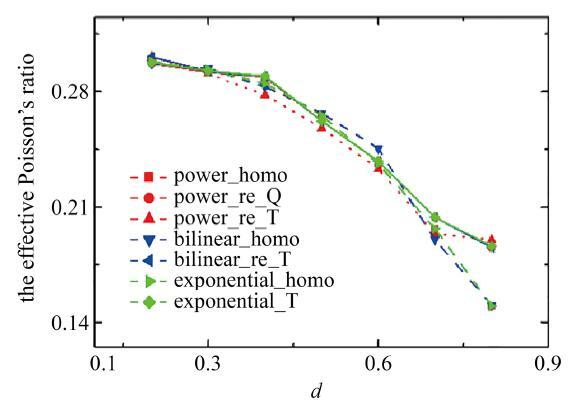Fig.5   The equivalent Poisson’s ratio of porous material structure

## 3 多尺度均匀化

### 3.1 玻璃纤维芯铅丝(网)增强橡胶复合材料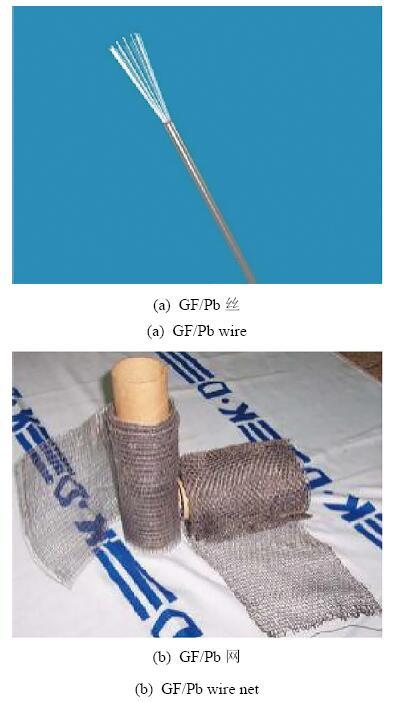Fig.6   GF/Pb wirebib21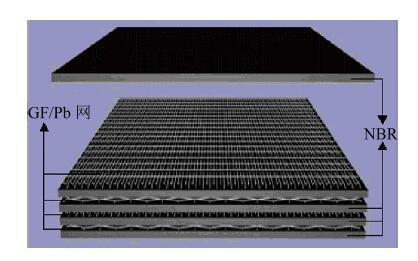Fig.7   Ply scheme of GF/Pb wire mesh and rubberbib21

### 3.2 芯丝尺度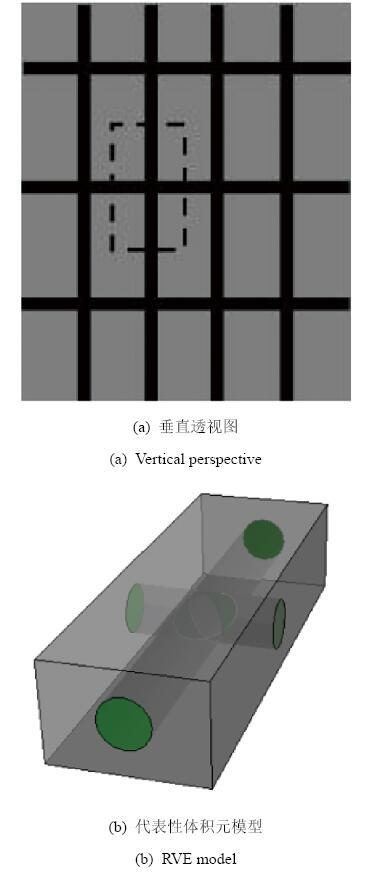Fig.8   GF/Pb wire geometric model at core-scale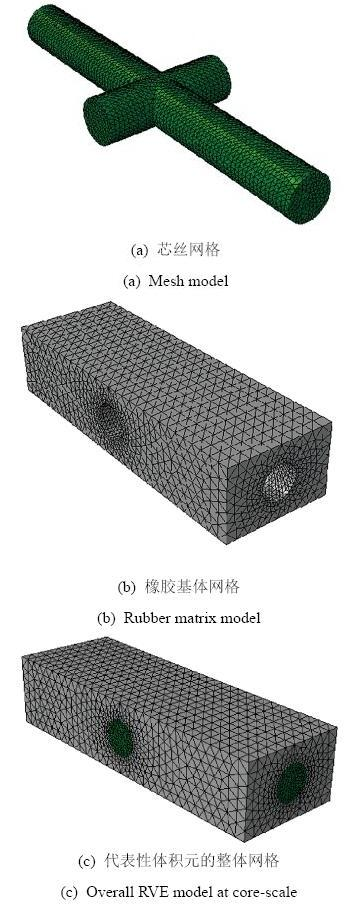Fig.9   RVE model at core-scale

### 3.3 层间尺度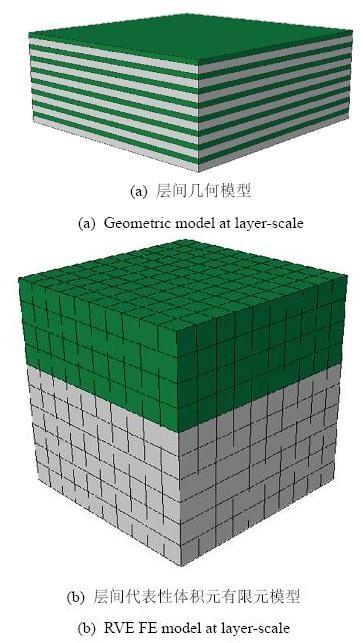Fig.10   Layer-scale model

### 3.4 多尺度均匀化方法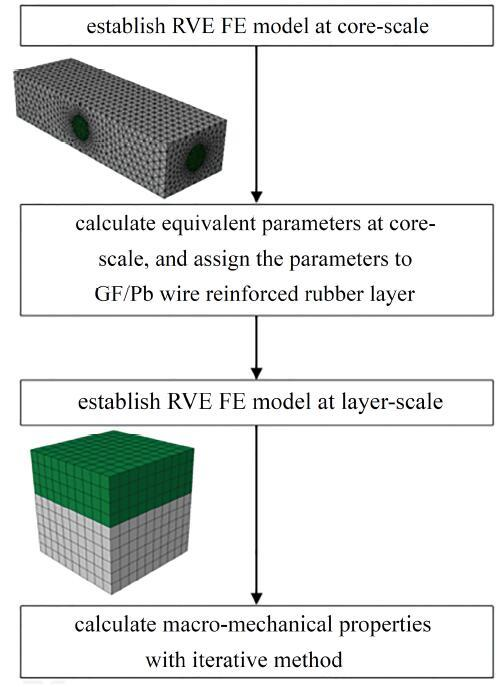Fig.11   Flow chart of multi-scale homogenization

(a) 在芯丝尺度下, 通过计算代表性体积元在单位应变下的位移, 得到其等效的弹性系数$Dijkl$. 所得到的弹性参数, 作为GF/Pb网增强橡胶薄层的力学性能参数赋给层间尺度, 将GF/Pb网增强橡胶薄层视为一种均匀分布的横观各向同性材料.

(b) 在层间尺度下, 将芯丝尺度的等效参数作为GF/Pb网增强橡胶薄层材料参数. 层间尺度涉及非线性弹性, 只能针对特定的载荷条件给出特定的宏观特性结果, 如最大位移、泊松比、应变能等. 经过迭代收敛得到的弹性模量, 可以作为该种载荷下材料整体性能的参考. 3.5多尺度均匀化结果

Table 2   The comparison among the equivalent parameters and the experimental data

Equivalent2*HomogenizationExperimental
$Ex$61.4357.60
$Ey$22.8121.20
$Ez$10.489.46
$μxy$0.470.45
$μyz$0.460.45
$μxz$0.430.45
$Gxy$2.572.36
$Gyz$3.242.36
$Gxz$3.022.36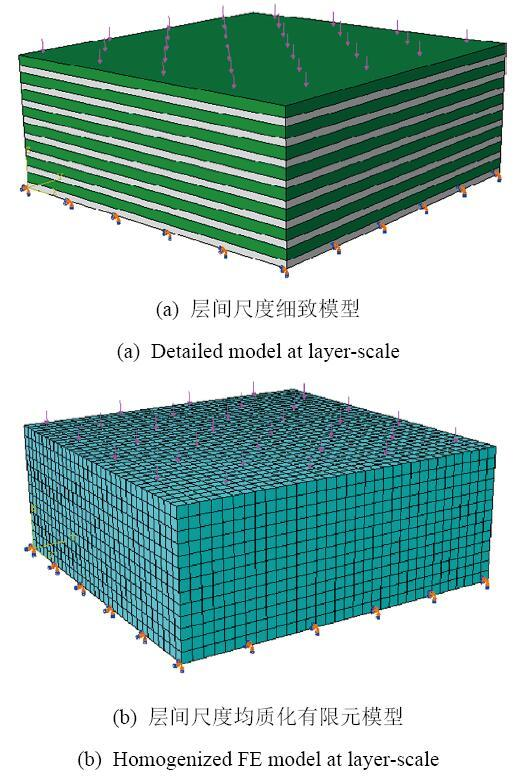Fig.12   Layer-scale model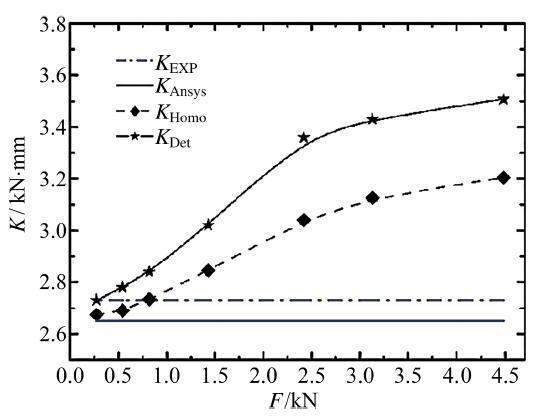Fig.13   The comparisons among the stiffness obtained by different layer-scale models

## 4 结论

(1) 非线性弹性均质化方法对于平面二维问题而言, 具有很好的精度. 均质化结构的应变能、最大位移和等效泊松比同细致模型的结果误差基本上保持在10$%$之内.

(2) 在变形较小时, 非线性弹性均质化方法在多尺度下依然可以较为准确的预测材料的宏观力学性能. 尽管由于缺少实验数据, 无法说明较大变形的精度, 但是从变化趋势上来说与理论上是吻合.

The authors have declared that no competing interests exist.

## 参考文献 原文顺序 文献年度倒序 文中引用次数倒序 被引期刊影响因子

  Eric L, Zhongpu Z, Chang CC, et al.Numerical homogenization for incompressible materials using selective smoothed finite element method.Composite Structures, 2015, 123: 216-232  朱合华, 陈庆. 多相材料有效性能预测的高精度方法. 力学学报, 2017, 49(1): 41-47 (Zhu Hehua, Chen Qing.An approach for predicting the effective properties of multiphase composite with high accuracy.Chinese Journal of Theoretical and Applied Mechanics, 2017, 49(1): 41-47 (in Chinese))  付云伟, 倪新华, 刘协权等. 颗粒缺陷相互作用下复合材料的细观损伤模型. 力学学报, 2016, 48(6): 1334-1342 (Fu Yunwei, Ni Xinhua, Liu Xiequan, et al.Micro-damage model of composite materials with particle and defect interaction.Chinese Journal of Theoretical and Applied Mechanics, 2016, 48(6): 1334-1342 (in Chinese))  Hashin Z.Analysis of composite materials.Journal of Applied Mechanics, 1983, 50(2): 481-505  Capdeboscq Y, Kang H.Improved Hashin-Shtrikman bounds for elastic moment tensors and an application.Applied Mathematics ＆ Optimization, 2008, 57(2): 263-288  Zimmerman R W.Hashin-Shtrikman bounds on the Poisson ratio of a composite material.Mechanics Research Communications, 1992, 19(6): 563-569  Mura T.Micromechanics of Defects in Solids. Germany: Springer Science ＆ Business Media, 2013: 10-53  张庆华, 刘西拉, 朱振宇. 用自洽方法计算混凝土的弹性模量.上海交通大学学报, 2001(10): 1503-1506 (Zhang Qinghua, Liu Xila, Zhu Zhengyu.Applying self-consistent method to calculate concrete modulus.Journal of Shanghai Jiaotong University, 2001(10): 1503-1506 (in Chinese))  Nasution MRE, Watanabe N, Kondo A et al. A novel asymptotic expansion homogenization analysis for 3-D composite with relieved periodicity in the thickness direction.Composites Science and Technology, 2014, 97(16): 63-73  魏志刚, 汤文成. 复合材料网格结构模态分析的均匀化等效建模. 复合材料学报, 2008(2): 188-193 (Wei Zhigang, Tang Wencheng.Homogenization modeling of composite lattice structure for mode analysis.Acta Materiae Compositae Sinica, 2008(2): 188-193 (in Chinese))  王飞, 庄守兵, 虞吉林. 用均匀化理论分析蜂窝结构的等效弹性参数.力学学报, 2002, 36(6): 914-923 (Wang Fei, Zhuang Shoubing,Yu Jilin. Application of homogenization FEM to the equivalent elastic constants of honeycomb structures.Acta Mechanica Sinica, 2002, 36(6): 914-923 (in Chinese))  Pinho J, Oliveira JA, Teixeira-Dias F.Asymptotic homogenization in linear elasticity. Part I: Mathematical formulation and finite element modeling.Computational Materials Science, 2009, 45(4): 1073-1080  Doghri I, Ouaar A.Homogenization of two-phase elasto-plastic composite materials and structures:<inline-formula><mml:math xmlns:mml="http://www.w3.org/1998/Math/MathML" id="Mml138-0459-1879-50-4-837"><mml:mi mathvariant="normal"> </mml:mi></mml:math></inline-formula>study of tangent operators, cyclic plasticity and numerical algorithms.International Journal of Solids and Structures, 2003, 40(7): 1681-1712  Andrianov IV, Danishevs Kyy VV, Ryzhkov OI, et al.Dynamic homogenization and wave propagation in a nonlinear 1D composite material.Wave Motion, 2013, 50(2): 271-281  Hoang TH, Guerich M, Yvonnet J.Determining the size of RVE for nonlinear random composites in an incremental computational homogenization framework.Journal of Engineering Mechanics, 2016, 142(5): 1-21  Cheng G, Cai Y, Xu L.Novel implementation of homogenization method to predicteffective properties of periodic materials.Acta Mechanica Sinica, 2013, 29(4): 550-556  沈观林, 胡更开, 刘彬. 复合材料力学. 第2版. 北京: 清华大学出版社, 2013: 48-52 (Shen Guanlin, Hu Gengkai, Liu Bin.Mechanics of Composite Materials. Second Edition. Beijing: Tinghua University Press, 2013: 48-52 (in Chinese))  龙述尧. 计算力学. 第1版. 长沙: 湖南大学出版社, 2007: 92-186 (Long Shurao. Computational Mechanics.The first edition. Changsha: Hunan University Press, 2007: 92-186 (in Chinese))  黄小双, 彭雄奇, 张必超. 帘线/橡胶复合材料各向异性黏-超弹性本构模型. 力学学报, 2016, 48(1): 140-145 (Huang Xiaoshuang, Peng Xiongqi, Zhang Bichao.An anisotropic visco-hyperelastic constitutive model for cord-rubber composites.Chinese Journal of Theoretical and Applied Mechanics, 2016, 48(1): 140-145 (in Chinese))  吕丽, 白书欣, 张虹等. 玻璃纤维芯铅丝增强橡胶复合材料阻尼性能研究.材料科学与工程学报, 2006(6): 843-846 (Lü Li, Bai Shuxin, Zhang Hong, et al.Damping property of glass-fiber-cored lead-wire reinforced rubber composite.Journal of Materials Science and Engineering, 2006(6): 843-846 (in Chinese))  吕丽. 玻璃纤维芯铅网(丝)增强橡胶阻尼复合材料性能研究: [博士论文]. 长沙: 国防科学技术大学, 2006:70-151 (Lü Li.The mechanical and damping properties of glass-fiber-net and glass-fiber-wire reinforced rubber composites. [PhD Thesis]. Changsha: National University of Defense Technology, 2006: 70-151 (in Chinese))  程哲, 张正艺, 宋杨等. 橡胶材料超弹性本构模型的简化标定方法. 固体力学学报, 2010(s1): 50-53 (Cheng Zhe, Zhang Zheng yi, Song Yang, et al. A simplified calibration method for the elastic constitutive model of rubber material. Acta Mechanica Solida Sinica#,2010(s1): 50-53 (in Chinese))/

 〈〉